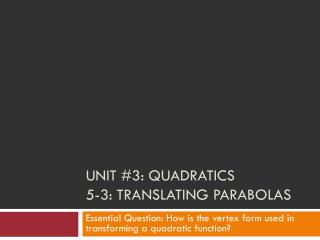DownloadDownload PresentationUnit #3: Quadratics 5-3: Translating Parabolas

# Unit #3: Quadratics 5-3: Translating Parabolas

Télécharger la présentation## Unit #3: Quadratics 5-3: Translating Parabolas

- - - - - - - - - - - - - - - - - - - - - - - - - - - E N D - - - - - - - - - - - - - - - - - - - - - - - - - - -
##### Presentation Transcript

1. Unit #3: Quadratics5-3: Translating Parabolas Essential Question: How is the vertex form used in transforming a quadratic function?

2. 5-3: Translating Parabolas • Yesterday, we explored quadratic equations in polynomial form: y = ax2 + bx + c • Two other forms which are used are x-intercept (factored) form and vertex (transformation) form. • Vertex form of a quadratic function is an equation in the form: y = a(x – h)2 + k • Just like in polynomial form: • If a is positive, the graph opens up • If a is negative, the graph opens down

3. 5-3: Translating Parabolas • y = a(x – h)2 + k • The vertex is found at (h, k) • h: Set what’s inside the parenthesis = 0 and solve for x • k: Take the number that’s being added to the end of the function. • Example • Find the vertex of y = (x + 3)2 – 1 • Because a = 1 (nothing in front of the parenthesis): • The graph opens up. • x + 3 = 0 → subtract 3 on each side →x = -3 • h = -3, k = -1 • The vertex is at (-3, -1).

4. 5-3: Translating Parabolas • Determine the vertex of the quadratic function and whether the graph opens up or down. • y = -3(x + 2)2 + 4 • Opens: • Vertex: • y = 2(x – 2.5)2 – 5.5 • Opens: • Vertex: • y = -½(x – 2)2 • Opens: • Vertex: Down (-2, 4) Up (2.5, -5.5) Down (2, 0)

5. 5-3: Translating Parabolas • To find the y-intercept: • Simply substitute “0” in for x and simplify • Example: y = -2(x – 3)2 + 4 • y = -2(0 – 3)2 + 4 • = -2(-3) 2 + 4 • = -2(9) + 4 • = -18 + 4 • = -14 • The y-intercept is at (0, -14)

6. 5-3: Translating Parabolas • To convert into polynomial form: • FOIL the parenthesis • DISTRIBUTE (if necessary) the number outside • COMBINE like terms • Example: y = -2(x – 3)2 + 4 • y = -2(x – 3)2 + 4 • = -2(x – 3)(x – 3) + 4 • = -2(x2 – 6x + 9) + 4 • = -2x2 + 12x – 18 + 4 • y = -2x2 + 12x – 14

7. 5-3: Translating Parabolas • Determine the y-intercept of the quadratic function and convert the function into polynomial form. • y = -3(x + 2)2 + 4 • y-intercept: • Polynomial form: • y = (x – 3)2 – 4 • y-intercept: • Polynomial form: • y = -½(x – 2)2 • y-intercept: • Polynomial form: (0, -8) y = -3x2 – 12x – 8 (0, 5) y = x2 – 6x + 5 (0, -2) y = -½x2 + 2x – 2

8. 5-3: Translating Parabolas • Assignment • Page 251 • Problems 1-11, odd • Ignore the directions!!! • Tell me: • Whether the graph opens up or opens down • The vertex • The y-intercept • The function written in polynomial form Note: You may want to do part (d) before part (c) • Tomorrow, Practice with graphing • Friday, Quiz on 5-1 through 5-3